## Overview

The model uses a finite difference approach to solve both electron and hole drift-diffusion equations in position space to describe the movement of charge within the device. At each mesh point in position space, a set of carrier trapping and escape equations are solved in energy space. Recombination can occur via a free-to-free (Langevin) recombination or by free-to-trap Shockley-Read-Hall type recombination. This approach enables the carrier population to be resolved in both position and energy space (see Figure 1)

Figure 1: Trap filling in both energy and position space as the solar cell is taken from a negative bias

## Carrier trapping, de-trapping, and recombination

In energy space the model describes the interaction of free carriers with a distribution of trap states using Shockley-Read-Hall (SRH) theory. A 0D section of the model is depicted in figure 2, the free electron and hole carrier distributions are labeled as n free and p free respectively. The trapped carrier populations are denoted with n trap and p trap , they are depicted with filled red and blue boxes. SRH theory describes the rates at which electrons and holes become captured and escape from the carrier traps. If one considers a single electron trap, the change in population of this trap can be described by four carrier capture and escape rates as depicted in figure 2. The rate rec describes the rate at which electrons become captured into the electron trap, ree is the rate which electrons can escape from the trap back to the free electron population, rhc is the rate at which free holes get trapped and rhe is the rate at which holes escape back to the free hole population. Recombination is described by holes becoming captured into electron space slice through our 1D traps. Analogous processes are also defined for the hole traps.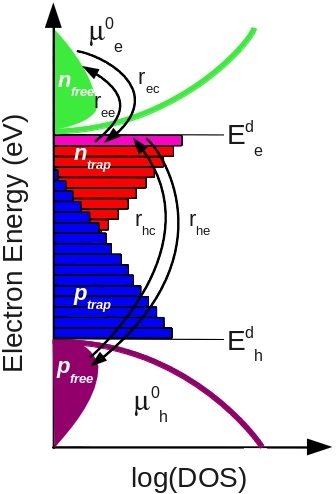Figure 2: A 0D energy slice through the model.

For each trap level the carrier balance equationis solved, giving each trap level an independent quasi-Fermi level. Each point in position space can be allocated between 10 and 160 independent trap states.

## Transport

To obtain the internal potential distribution within the device Poisson's equation is solved,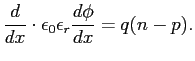The bi-polar drift diffusion equations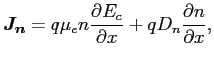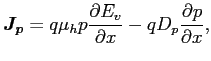And the carrier continuity equations in 1D and in time domain.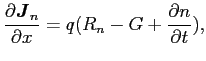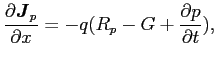## Solving the equations

The above set of non linear equations are placed into a single sparse matrix and solved. The drift diffusion are cast into a Scharfetter-Gummel form, to ensure stability.

## The optical problem

There are three optical model available:
1. A model which assumes the distributions of photons in the device is flat and uniform.
2. A model which assumes light intensity decays exponentially as it penetrates the device.
3. A standard transfer matrix approach where the forward and backward propagating waves are solved, taking into account reflections at interfaces.